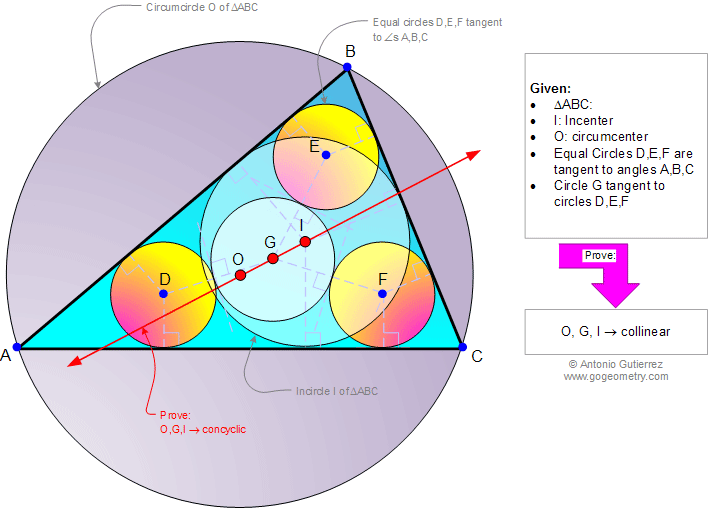# Online Geometry Problem 952: Triangle, Incenter, Circumcenter, Three Equal Circles, Tangent Line, Tangent Circles, Collinear Points. Level: High School, SAT Prep, College, Mathematics Education

< PREVIOUS PROBLEM  |  NEXT PROBLEM >

 The figure below shows a triangle ABC with the circumcenter O and incenter I. Equal circles D, E, and F are tangent to angles A, B, and C, respectively. Circle G is tangent to circles D, E, and F. Prove that points O, G, and I are collinear.Home | Search | Geometry | Problems | All Problems | Open Problems | Visual Index | 10 Problems | 951-960 | Triangles | Circles | Angle | Tangent Circles | Circle Tangent Line | Circumcenter | Incenter | Email | Solution / comment | By Antonio Gutierrez